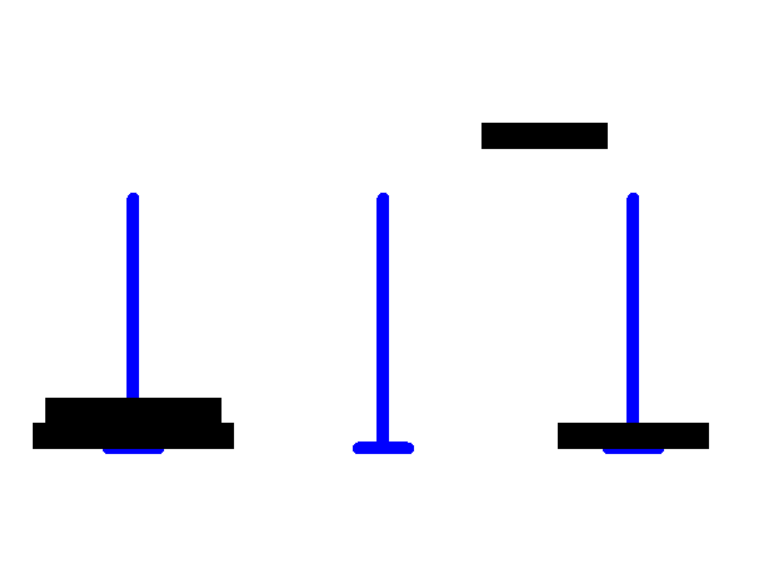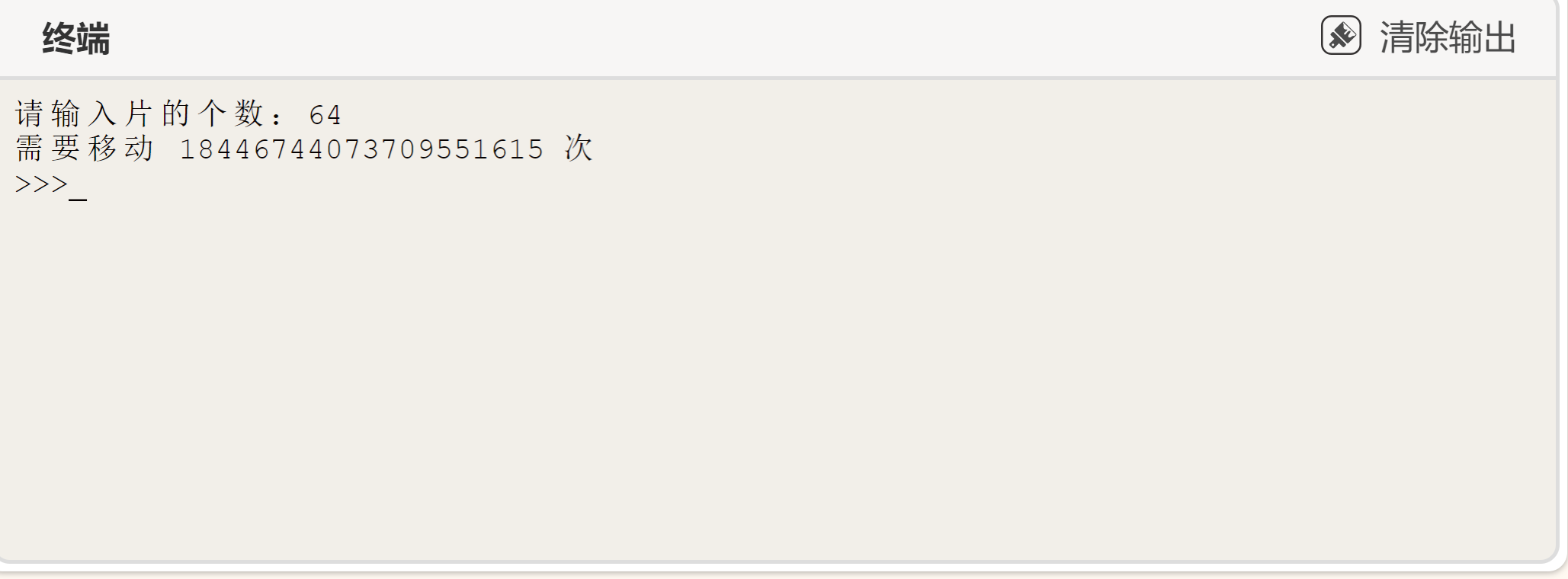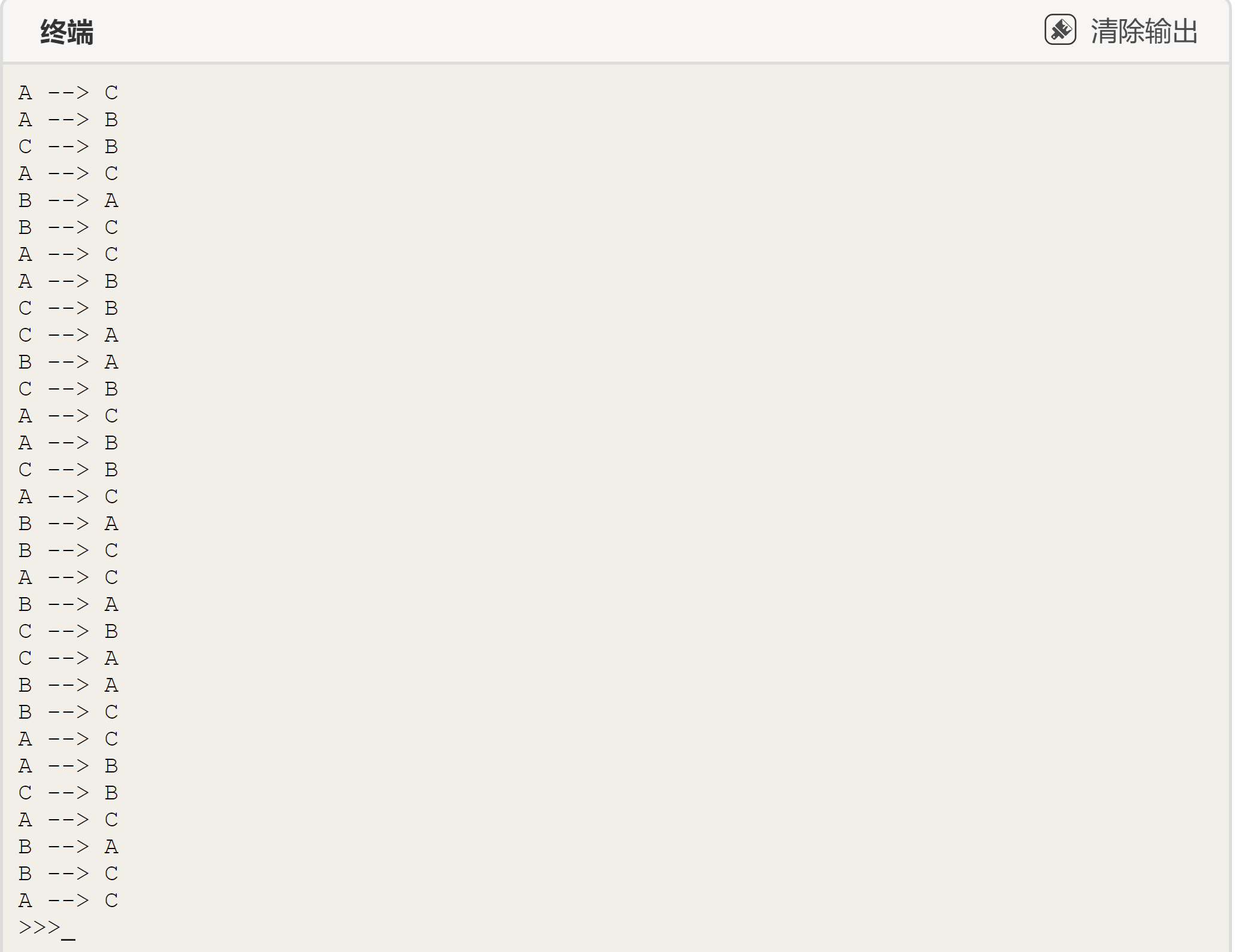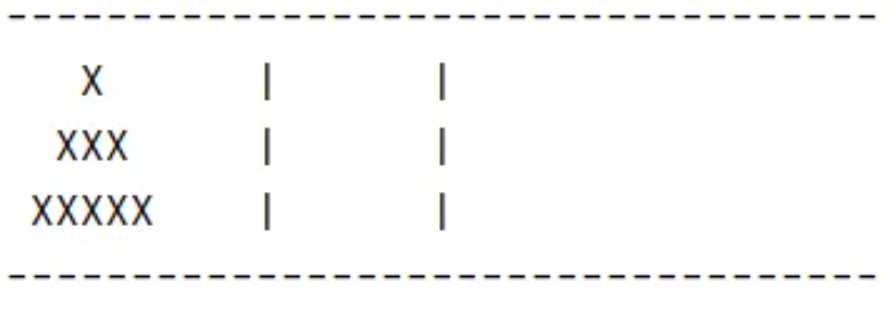[[d.project_title]][[d.material_name]]# 大家快和我使用Mind+写一个属于自己的小游戏吧！（汉诺塔）```	                    					```def f(n):
if n==0:
return 0
else:
return 2*f(n-1)+1
x=int(input("请输入片的个数："))
print("需要移动",f(x),"次")```
```⑴按顺时针方向把圆盘1从现在的柱子移动到下一根柱子，即当n为偶数时，若圆盘1在柱子A，则把它移动到B；若圆盘1在柱子B，则把它移动到C；若圆盘1在柱子C，则把它移动到A。

⑵接着，把另外两根柱子上可以移动的圆盘移动到新的柱子上。即把非空柱子上的圆盘移动到空柱子上，当两根柱子都非空时，移动较小的圆盘。这一步没有明确规定移动哪个圆盘，你可能以为会有多种可能性，其实不然，可实施的行动是唯一的。

⑶反复进行⑴⑵操作，最后就能按规定完成汉诺塔的移动。

```	                    					```def hanoi(n, a, b, c):
if n == 1:
print(a, '-->', c)
else:
hanoi(n - 1, a, c, b)
print(a, '-->', c)
hanoi(n - 1, b, a, c)
# 调用
hanoi(5, 'A', 'B', 'C')```
``````	                    					```left = list()
center = list()
right = list()
```
```

```	                    					```def init():
"""
初始化函数
"""
size = input("(请输入整数)请输入层数:")
# 初始化塔列表,如5层 左边塔放 1-3-5-7-9,中间和右边放5个-1
for i in range(1, int(size) + 1):
left.append(i*2-1)
center.append(-1)
right.append(-1)
return int(size)```
```

```	                    					```def printStyling(i, size, ta):
"""
打印样式函数
"""
if ta[i] != -1:
# 打印前空格
for kong in range(int(size - (ta[i] - 1) / 2)):
print(" ", end="")
# 打印塔元素
for le in range(ta[i]):
print("X", end="")
# 打印后空格
for kong in range(int(size - (ta[i] - 1) / 2)):
print(" ", end="")
# 左塔这一层为空格
else:
# 打印前面空格
for kong in range(size):
print(" ", end="")
# 打印中间的棒棒
print("|", end="")
# 打印后面的空格
for kong in range(size):
print(" ", end="")```
``````	                    					```def show(size):
"""控制台打印结果"""
# 修饰
print("-"*35)
# 循环层数等于size
for i in range(size):
# 打印左边塔
printStyling(i, size, left)
# 打印中间塔
printStyling(i, size, center)
# 打印右边塔
printStyling(i, size, right)
# 每行打印一个换行
print()
# 修饰
print("-" * 35)```
```

```	                    					```def judge(takeOff, putOn, size, tSize, pSize, count):
"""判断可不可以移动
takeOff减少,putOn增加,size层数,tSize和pSize剩余空间
"""
# 如果左塔的空间空的,就是没有元素可移动
if takeOff == size:
print("操作无效!")
return 0
# 如果中塔为空,可以移动
if pSize == size:
# 中间的最后一个元素赋上左塔的第一个元素的值
putOn[pSize - 1] = takeOff[tSize]
# 左塔的第一个元素赋值-1
takeOff[tSize] = -1
# 左塔的剩余空间+1
tSize += 1
# 中塔的剩余空间-1
pSize -= 1
# 步数+1
count += 1
# 移动成功,返回剩余空间和步数
return tSize, pSize, count
# 如果中塔最上方元素比左塔最上方元素大,即可以移动
elif putOn[pSize] > takeOff[tSize]:
# 中塔当前最上方元素的再上一个元素(-1)赋上左塔最上方元素的值
putOn[pSize - 1] = takeOff[tSize]
#  左塔最上方元素赋值-1
takeOff[tSize] = -1
# 左塔剩余空间+1
tSize += 1
# 中塔剩余空间-1
pSize -= 1
# 数+1
count += 1
# 移动成功,返回剩余空间和步数
return tSize, pSize, count
# 否则不可以移动
else:
print("操作无效!")
return 0```
```

```	                    					```def main():
"""

"""
# 初始化游戏
size = init()
# 存放最初的盘剩余空间 lSize左塔 cSize中塔 rSize
lSize = 0
cSize = size
rSize = size
# 存放操作步数
count = 0
# 打印游戏介绍
print("将左塔完整地移到右塔就是胜利!")
print("左-1 中-2 右-3 退出请输入:quit")
print('例如输入:"1-2"就是将左塔的最上元素放到中
print("%d层的最佳步数是%d" % (size, pow(2, size)
# 游戏进行
while True:
print("当前移动了%d步" % (count))
# 显示当前塔的状态
show(size)
# 判断右塔是否没有剩余空间,没有即胜利,并退出
if rSize == 0:
if count == pow(2, size)-1:
print("恭喜你使用最少步数完成汉诺塔!
else:
print("恭喜你只移动了%d步完成汉诺塔
break
# 获取玩家操作
select = input("请操作:")
# 左塔移中塔
if select == "1-2":
result = judge(left, center, size, lSize
if result == 0:
continue
else:
lSize, cSize, count = result
# 左塔移右塔,下面同样
elif select == "1-3":
result = judge(left, right, size, lSize,
if result == 0:
continue
else:
lSize, rSize, count = result
elif select == "2-1":
result = judge(center, left, size, cSize
if result == 0:
continue
else:
cSize, lSize, count = result
elif select == "2-3":
result = judge(center, right, size, cSiz
if result == 0:
continue
else:
cSize, rSize, count = result
elif select == "3-1":
result = judge(right, left, size, rSize,
if result == 0:
continue
else:
rSize, lSize, count = result
elif select == "3-2":
result = judge(right, center, size, rSiz
if result == 0:
continue
else:
rSize, cSize, count = result
# 输入quit退出游戏
elif select == "quit":
break
# 如果输入的是其他不识别的文字,就拜拜
else:
print("操作有误!")
continue
main()
```
```

Makelog作者原创文章，未经授权禁止转载。
1
0[[c.user_name]] [[c.create_time]]
[[c.parent_comment.count]]
|
[[c.comment_content]]
[[r.user.user_name]] [[r.create_time]]
[[r.comment_content]]

0
1
0
2
3
11
1
3
2
5